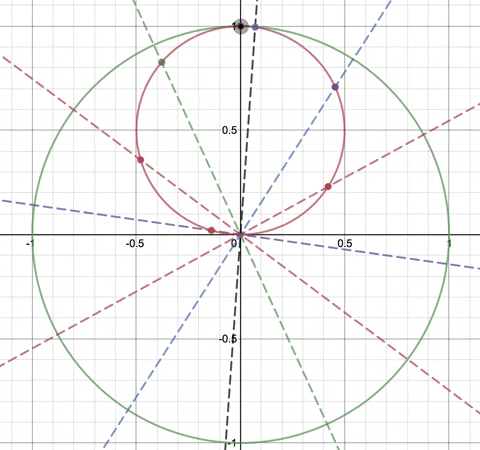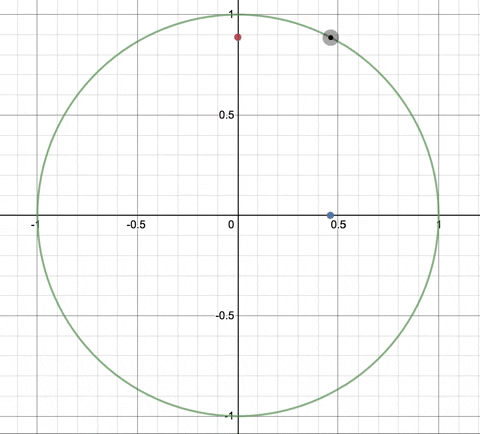You are currently browsing the tag archive for the ‘Tusi couple’ tag.The Tusi couple – A circle rolling inside a circle

Numberphile have done a nice video where they discuss some beautiful examples of trigonometry and circular motion and where they present the result shown above: a circle rolling within a circle, with the individual points on the small circle showing linear motion along the diameters of the large circle.  So let’s see what maths we need to create the image above.

Projection of pointsWe can start with the equation of a unit circle centred at the origin:and we can then define a point on this circle parametrically by the coordinate:Here t is the angle measured from the horizontal.

If we then want to see the projection of this point along the y-axis we can also plot:and to see the projection of this point along the x-axis we can also plot:By then varying t from 0 to 2 pi gives the animation above – where the black dot on the circle moves around the circle and there is a projection of its x and y coordinates on the axes.

Projection along angled linesI can then add a line through the origin at angle a to the horizontal:and this time I can project so that the line joining up the black point on the edge of the large circle intersects the dotted line in a right angle.

In order to find the parametric coordinate of this point projection I can use some right angled triangles as follows:The angle from the horizontal to my point A is t.  The angle from the horizontal to the slanted line is a.  The length of my radius BA is 1.  This gives me the length of BC.But I have the identity:Therefore this gives:And using some more basic trigonometry gives the following diagram:Therefore the parametric form of the projection of the point can be given as:I can add several more slanted lines through the origin.  You can see that each dot on the line is the right angle projection between the line and the point on the circle.  As we do this we can notice that the points on the lines look as though they form a circle.  By noticing that the new smaller circle is half the size of the larger circle, and that the centre of the smaller circle is half-way between the origin and the point on the large circle, we get:We can the vary the position of the point on the large circle to then create our final image:We have a connection between both linear motion and circular motion and create the impression of a circle rolling inside another.

You can play around with this demos graph here.  All you need to do is either drag the black point around the circle, or press play for the t slider.

More ideas on projective geometry:Ferenc Beleznay has made this nice geogebra file here which shows a different way of drawing a connection between a moving point on a large circle and a circle half the size. Here we connect the red dot with the origin and draw the perpendicular from this line to  the other edge of the small circle.  The point of intersection of the two lines is always on the small circle.

Further exploration

There is a lot more you can explore – start by looking into the Tusi Couple – which is what we have just drawn – and the more general case the hypocycloid.Exploration Guide

A comprehensive 70 page pdf guide to help you get excellent marks on your maths investigation. Includes how to choose a topic, over 70 topic ideas, marking criteria guidance, Pearson's Product method, common students mistakes, in-depth topic examples and much more! [Will be emailed within the same day as ordered].

\$5.50

### Website Stats

• 7,158,943 views